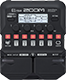# Doom metal

Discussion in 'Zoom G1/G1X Four' started by jes, Apr 25, 2021.

1. Doom metalDevice: Zoom G1 Four
Firmware: 2.00

Name on device: MelodicMtl
Optimized for: Phones/Speaker

Effects chain:Like Swallow the sun, Insomnium. Less mids, more lows, some mid and highs

Effect: "ZNR" (Dynamics), active - "yes"
"Detect" = EFXIN
"Depth" = 79
"Threshold" = 74
"Decay" = 20

Effect: "MetalWRLD" (Overdrive / Distortion), active - "yes"
"Gain" = 88
"Bass" = 100
"Treble" = 38
"Volume" = 100

Effect: "DIST Plus" (Overdrive / Distortion), active - "yes"
"Gain" = 86
"Volume" = 84
"DryMx" = 40
"Comp" = ORG

Effect: "Gt GEQ 7" (Filter), active - "yes"
"100" = 4.0
"200" = 7.0
"400" = 7.5
"800" = -5.0
"1.6k" = -7.5
"3.2k" = 5.5
"6.4k" = 1.0
"VOL" = 100

Effect: "Air" (Reverb), active - "yes"
"Size" = 20
"REF" = 7
"Mix" = 72
"Tail" = Off
Patch Volume: 73